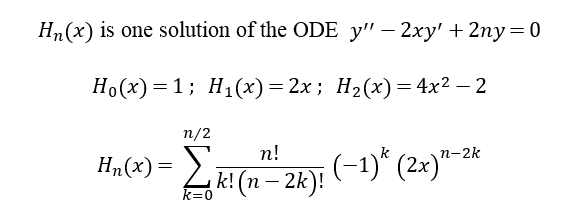Hermite Polynomials !

Enter Order of the Polynomial (< 61)

Hermite polynomials are solutions to a specific, linear, second-order ordinary differential equation (ODE) with a non-negative integer parameter called the "order." Thus, the Hermite polynomial of order n is one solution to the Hermite ODE with this parameter n. The zeroes of each Hermite polynomial are critically important to an ingenius numerical integration technique called Gauss-Hermite Quadrature. We calculate these zeroes as well as the corresponding "weights" for the quadrature method in this web app. We show only the non-negative zeroes (and corresponding weights). The negative of each positive zero is also an Hermite zero and shares the same weight as its positive counterpart. See this quantitative finance article and this simple, yet elaborate, explanation of Hermite Polynomials and Gauss Quadrature. We also found Greenwood and Miller (1948) to be helpful.

Non-Negative Zeroes GHQ Weights Non-Negative Zeroes GHQ Weights